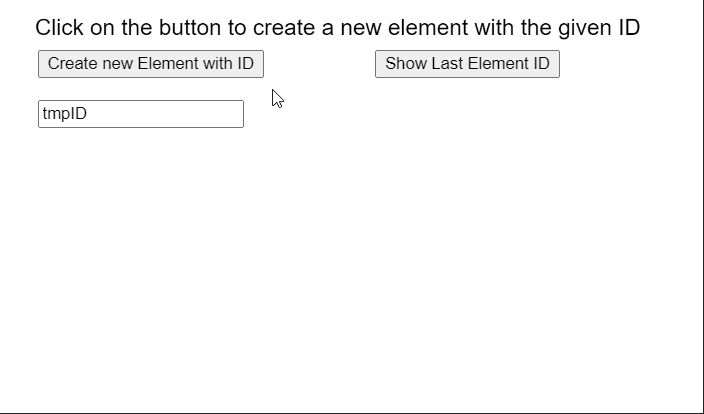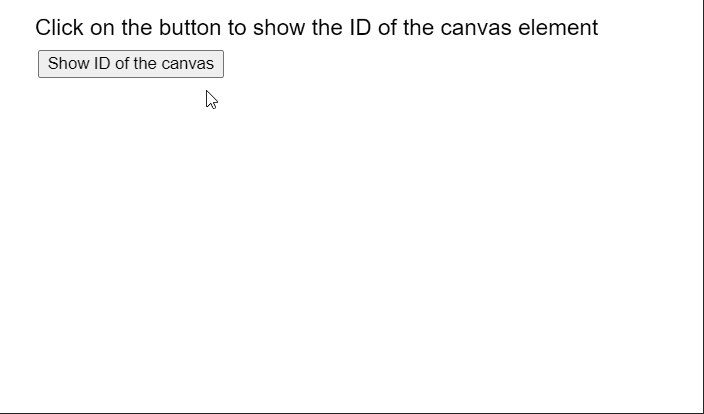Related Articles

# p5.js Element id() Method

• Last Updated : 18 Nov, 2020

The id() method of p5.Element in p5.js is used to set or return the ID of an element. When no ID is specified as a parameter, it returns the current ID of the element. Only one element on the page can have a particular ID specified to it.

Syntax:

```id( id )
```

Parameters: This function accepts a single parameter as mentioned above and described below.

• id: It is a string that denotes the ID of the element.

Example 1: The example below illustrates the id() method in p5.js.

## Javascript

 `function` `setup() {``  ``createCanvas(550, 300);``  ``textSize(18);`` ` `  ``text(``"Click on the button to create a new "` `+``       ``"element with the given ID"``, 20, 20);`` ` `  ``setBtn = ``    ``createButton(``"Create new Element with ID"``);``  ``setBtn.position(30, 40);``  ``setBtn.mouseClicked(createNewElement);`` ` `  ``setBtn = ``    ``createButton(``"Show Last Element ID"``);``  ``setBtn.position(300, 40);``  ``setBtn.mouseClicked(showID);`` ` `  ``id_name = createInput(``'tmpID'``);``  ``id_name.position(30, 80);``}`` ` `function` `createNewElement() {``  ``clear();`` ` `  ``// Create a new p5.Element``  ``tmpElement = createElement(``"p"``);`` ` `  ``// Get the ID to set``  ``let idToSet = id_name.value();`` ` `  ``// Set the ID of the element``  ``tmpElement.id(idToSet);`` ` `  ``text(``"New element created with ID: "` `+``       ``idToSet, 30, 120);`` ` `  ``text(``"Click on the button to create a new "` `+``       ``"element with the given ID"``, 20, 20);``}`` ` `function` `showID() {``  ``clear();`` ` `  ``// Get the ID of the element``  ``let setID = tmpElement.id();`` ` `  ``// Display the ID``  ``text(``"The ID of the last element is: "` `+ ``       ``setID, 30, 120);`` ` `  ``text(``"Click on the button to create a new "` `+``       ``"element with the given ID"``, 20, 20);``}`

Output:Example 2:

## Javascript

 `function` `setup() {`` ` `  ``// Create a new canvas``  ``canv = createCanvas(550, 300);`` ` `  ``// Set the ID of the canvas``  ``canv.id(``"newCanvas"``);`` ` `  ``textSize(18);``  ``text(``"Click on the button to show the ID "` `+``       ``"of the canvas element"``, 20, 20);`` ` `  ``setBtn = ``    ``createButton(``"Show ID of the canvas"``);``  ``setBtn.position(30, 40);``  ``setBtn.mouseClicked(showID);``}`` ` `function` `showID() {``  ``clear();`` ` `  ``// Get the ID of the element``  ``let setID = canv.id();`` ` `  ``// Display the ID``  ``text(``"The ID of the canvas is: "` `+``       ``setID, 20, 80);`` ` `  ``text(``"Click on the button to show the ID "` `+``       ``"of the canvas element"``, 20, 20);``}`

Output:Online editor: https://editor.p5js.org/
Environment Setup: https://www.geeksforgeeks.org/p5-js-soundfile-object-installation-and-methods/
Reference: https://p5js.org/reference/#/p5.Element/id

My Personal Notes arrow_drop_up